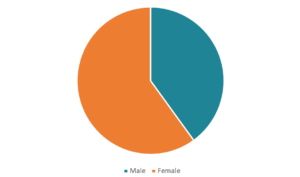# Nominal Data

Data that is used to label variables without providing quantitative values

## What is Nominal Data?

In statistics, nominal data (also known as nominal scale) is a type of data that is used to label variables without providing any quantitative value. It is the simplest form of a scale of measure. Unlike ordinal data, nominal data cannot be ordered and cannot be measured.Dissimilar to interval or ratio data, nominal data cannot be manipulated using available mathematical operators. Thus, the only measure of central tendency for such type of data is the mode.

### Characteristics of Nominal Data

Nominal data can be both qualitative and quantitative. However, the quantitative labels lack a numerical value or relationship (e.g., identification number). On the other hand, various types of qualitative data can be represented in nominal form. They may include words, letters, and symbols. Also, names of people, gender, and nationality are just a few of the most common examples of nominal data.

### How to Analyze Nominal Data?

Nominal data can be analyzed using the grouping method. The variables can be grouped together into categories, and for each category, the frequency or percentage can be calculated. The data can also be presented visually such as by using a pie chart.

Although nominal data cannot be treated using mathematical operators, they still can be analyzed using advanced statistical methods. For example, one way to analyze the data is through hypothesis testing.

For nominal data, hypothesis testing can be carried out using nonparametric tests such as the chi-squared test. The chi-squared test aims to determine whether there is a significant difference between the expected frequency and the observed frequency of the given values.

### More Resources

CFI offers the Financial Modeling & Valuation Analyst (FMVA)™ certification program for those looking to take their careers to the next level. To keep learning and advancing your career, the following resources will be helpful:

• Basic Statistics Concepts in Finance
• Independent Events
• Positively Skewed Distribution
• Quantitative Analysis

### Financial Analyst Certification

Become a certified Financial Modeling and Valuation Analyst (FMVA)® by completing CFI’s online financial modeling classes and training program!